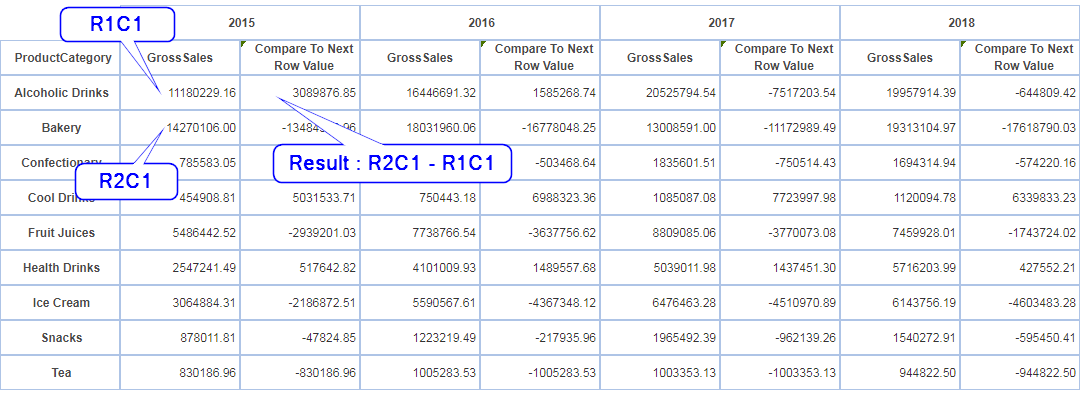1. Find out the difference in values between two rows

Description:

Find out the difference in values between two rows (R1 and R2) in the crosstab i.e.; the Relative Row difference, considering the column as the same (C1)

Example:As per the above figure, R1C1 value is 11180229.16 which is the Gross sales of ‘Alcoholic Drinks’ for the year ‘2015’.And R2C1 value is 14270106.00 which is the Gross Sales of ‘Bakery’ for the year ‘2015’. The result after the difference (R2C1 – R1C1) would be 14270106.00 – 11180229.16 = 3089876.85.

UDDC Expression:

 r2c1 - r1c1